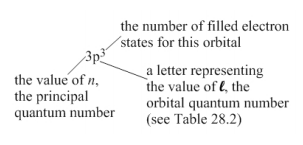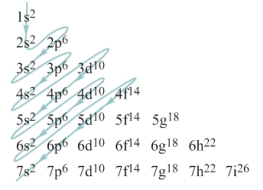Pauli’s Exclusion Principle

Pauli exclusion principle states that no two electrons can simultaneously occupy the same electron state in an atom. In other words, no two electrons in an atom can simultaneously have the same set of four quantum numbers.

Introduction To Pauli’s Exclusion Principle

The exclusion principle is named for the Austrian physicist Wolfgang Pauli (1900–1958). Pauli was awarded the Nobel Prize in Physics in 1945 for the exclusion principle. With the exclusion principle in mind, let’s examine the ground state (lowest energy) configurations of various atoms. In general, equation 28.3, in which the energy of an electron state is determined solely by the principal quantum number, is inadequate. The energy is also determined by the electron’s orbital angular momentum. In general, for a given value of the principal quantum number, n, the higher the value of the orbital quantum number, L, the higher the energy of that state.

Let’s begin by looking at the ground state configurations of a few elements in the periodic table. These are shown in Table 28.1. An explanation of what the number-letter-number notation means is given in Figure 28.8.

 Atomic number Name (symbol) Ground-state configuration 2 Helium (He) 1s2 6 Carbon (C) 1s2 2s2 2p2 10 Neon (Ne) 1s2 2s2 2p6 15 Phosphorus (P) 1s2 2s2 2p6 3s2 3p3 20 Calcium (Ca) 1s2 2s2 2p6 3s2 3p6 4s2 26 Iron (Fe) 1s2 2s2 2p6 3s2 3p6 4s2 3d4

Table 28.1: Ground-state configurations for selected atoms.Figure 28.8: Explaining the number-letter-number notation.

Referring to Table 28.1, we see that helium, with only two electrons, can have both electrons in the n = 1 shell, which can only fit two electrons. For atoms that have more than 2 electrons, two electrons are in the n = 1 level, and then the others are in states that have larger n values. The n = 2 level can fit eight electrons (two with L = 0 and six with L = 1). Thus, for elements up to and including neon, which has 10 electrons in its ground-state configuration, the electrons are in the n = 1 and n = 2 levels. Beyond this, electron state with higher n values come into play.

The layout of the ground-state configurations in Table 28.1 needs further explanation. This is provided by Table 28.2, which shows what value of L the various letters correspond to, and by Figure 28.9, which shows how the various orbitals compare to one another, in terms of their energy level. As you wind your way through Figure 28.9 from top to bottom, the energy of particular orbital increases. This figure shows the order in which electrons fill states in the ground-state configuration because the ground-state configuration minimizes the total energy of the electrons.

 Value of L Letter (stands for) 0 s (sharp) 1 p (principal) 2 d (diffuse) 3 f (fundamental) 4 g (letter after f) 5 h (letter after g) 6 i (letter after h)

Table 28.2: Letters for various L values.Figure 28.9: The order in which electrons fill the different subshells.

Terminology

An atomic orbital is both the mathematical function that describes where an electron can be and the corresponding region in space. It takes three quantum numbers (n, L, and mL) to define an orbital. Because there are two possible values of the spin quantum number, each atomic orbital can contain two electrons.

A subshell is the set of orbitals with the same values of n and L.

A shell is the set of orbitals with the same value of n.

Figure 28.9 is consistent with what is known as the Aufbau principle, derived from the German word Aufbau Prinzip, which means “building-up principle.” The basic rule is that subshells are filled in order of lowest n + L values. In cases of equal n + L values, the subshells with the lower n value are filled first. The diagonal lines in Figure 28.9 represent subshells that have equal n + L values, with those with the lower n values coming first.

Practise This Question

When a magnet is moved quickly towards a closed coil the induced emf and charge are e' and q'.  When the same magnet is slowly towards the same coil, the induced emf and charge are e1 and q1  respectively.  Then